# Features of C++ 17

• Difficulty Level : Easy
• Last Updated : 20 Nov, 2021

C++17 enables writing simple, clearer, and more expressive code. Some of the features introduced in C++17 are:

• Nested Namespaces
• Variable declaration in if and switch
• if constexpr statement
• Structured bindings
• Fold Expressions
• Direct list initialization of enums

### Nested Namespaces

Namespaces are a very convenient tool to organize and to structure the code base, putting together components like classes and functions that logically belong to the same group.

Let’s consider a hypothetical code base of a video game engine. Here, defined a namespace for the whole game engine, so all the classes and the functions implemented in this Game Engine will be declared under this common namespace. To do more clear definitions you can define another namespace under the global namespace lets say Graphics which is a sub-namespace, now put all classes that perform graphics operations under that namespace and so on.

• Before C++17:
Below is the syntax used for nested namespace:

## C++

 `// Below is the syntax for using` `// the nested namespace`   `namespace` `Game {`   `    ``namespace` `Graphics {`   `        ``namespace` `Physics {`   `           ``class` `2D {` `              ``..........` `           ``};` `        ``}` `    ``}` `}`

• When C++17:

Before C++17 you have to use this verbose syntax for declaring classes in nested namespaces, but C++17 has introduced a new feature that makes it possible to open nested namespaces without this hectic syntax that require repeated namespace keyword and keeping track of opening and closing braces. In C++17 there a simple and concise syntax using double colons to introduce nested namespaces. The syntax is as follows:

## C++

 `// Below is the syntax to use the` `// nested namespace in one line`   `namespace` `Game::Graphics::Physics {`   `    ``class` `2D {` `       ``..........` `    ``};` `}`

This makes the code less error-prone as there is no need to pay attention to several levels of braces.

### Variable declaration in if and switch

Before C++17:

Suppose a vector of strings and you want to replace a string “abc” with “\$\$\$” if it is present in the vector. To do that you can invoke the standard find() function to search for an item in a vector as shown below:

## C++

 `// Below is the approach for replace` `// any string with another string` `// in vector of string`   `vector str`   `    ``// Find and replace abc with \$\$\$` `    ``const` `auto` `it` `    ``= find(begin(str), end(str), ``"abc"``);`   `    ``if` `(it != end(str)) {` `       ``*it = ``"\$\$\$"``;` `    ``}`

Explanation:

• The find algorithm will return an iterator pointing to the matched string.
• Now, if again we want to replace another string with some other string in the same vector, then for this, follow the same approach as shown above, and as you will repeat the same code to just have to change the name of the iterator to something else.
• As declaring more than two iterators with the same name in the same scope will give a compilation error. The name changing option will work but if there are several strings that need to be replaced, but this approach is not efficient.

When C++17:
For dealing with such cases C++17 gives a better option by declaring variables inside if statements as shown below:

## C++

 `// Below is the syntax for replacing a` `// string in vector of string in C++17`   `if` `(``const` `auto` `it = find(begin(str),` `                         ``end(str),` `                         ``"abc"``);` `    ``it != end(str)) {` `    ``*it = ``"\$\$\$"``;` `}`

Now the scope of the iterator “it” is within the if statement itself, and the same iterator name can be used to replace other strings too.
The same thing can be done using a switch statement to using the syntax given below:

```switch (initial-statement; variable) {
....
// Cases
}```

Below is the program that replaces some defined strings in the given vector that runs only in C++17:

## C++

 `// C++ 17 code to demonstrate if constexpr`   `#include ` `#include ` `#include ` `#include ` `using` `namespace` `std;`   `// Helper function to print content` `// of string vector` `void` `print(``const` `string& str,` `           ``const` `vector& vec)` `{` `    ``cout << str;` `    ``for` `(``const` `auto``& i : vec) {` `        ``cout << i << ``" "``;` `    ``}` `    ``cout << endl;` `}`   `// Driver Code` `int` `main()` `{` `    ``// Declare vector of string` `    ``vector vec{ ``"abc"``, ``"xyz"``,` `                        ``"def"``, ``"ghi"` `};`   `    ``// Invoke print helper function` `    ``print(``"Initial vector: "``, vec);`   `    ``// abc -> \$\$\$, and the scope of "it"`   `    ``// Function invoked for passing` `    ``// iterators from begin to end` `    ``if` `(``const` `auto` `it = find(begin(vec),` `                             ``end(vec), ``"abc"``);`   `        ``// Check if the iterator reaches` `        ``// to the end or not` `        ``it != end(vec)) {`   `        ``// Replace the string if an` `        ``// iterator doesn't reach end` `        ``*it = ``"\$\$\$"``;` `    ``}`   `    ``// def -> ###` `    ``// Replace another string using` `    ``// the same iterator name` `    ``if` `(``const` `auto` `it` `                 ``= find(begin(vec),` `                        ``end(vec), ``"def"``);`   `        ``it != end(vec)) {` `        ``*it = ``"###"``;` `    ``}` `    ``print(``"Final vector: "``, vec);` `    ``return` `0;` `}`

Output: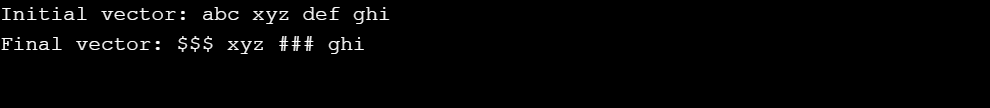### If constexpr statement

This feature of C++ 17 is very useful when you write template code. The normal if statement condition is executed at run time, so C++17 introduced this new if constexpr statement. The main difference is that if constexpr is evaluated at compile time. Basically, constexpr function is evaluated at compile-time. So why is this important, its main importance goes with template code.

Before C++17:
Suppose, to compare if an integer variable with a value, then declare and initialize that integer at compile time only before using that variable as shown below:

## C++

 `// Below is the syntax for using` `// If-else statement`   `int` `x = 5;`   `// Condition` `if` `(x == 5) {` `    ``// Do something` `}` `else` `{` `    ``// Do something else` `}`

When C++17:

Suppose you have some template that operates on some generic type T.

## C++

 `// Below is the generic code for` `// using If else statement`   `template` `<``typename` `T>`   `// Function template for illustrating` `// if else statement` `auto` `func(T ``const` `&value)` `{` `    ``if` `        ``constexpr(T is integer)` `        ``{` `            ``// Do something` `        ``}` `    ``else` `{` `        ``// Something else` `    ``}` `}`

So one aspect of constexpr is that now the compiler knows if T is an integer or not, and the compiler considers only the substatement that satisfies the condition so only that block of code is compiled and the C++ compiler ignores the other substatements.

Below is the program for the same:

## C++

 `// C++ 17 code to demonstrate error` `// generated using if statement`   `#include ` `#include ` `#include ` `using` `namespace` `std;`   `// Template Class` `template` `<``typename` `T>` `auto` `length(T ``const``& value)` `{`   `    ``// Check the condition with if` `    ``// statement whether T is an` `    ``// integer or not` `    ``if` `(is_integral::value) {` `        ``return` `value;` `    ``}` `    ``else` `{` `        ``return` `value.length();` `    ``}` `}`   `// Driver Code` `int` `main()` `{` `    ``int` `n{ 10 };`   `    ``string s{ ``"abc"` `};`   `    ``cout << ``"n = "` `<< n` `         ``<< ``" and length = "` `         ``<< length(n) << endl;`   `    ``cout << ``"s = "` `<< s` `         ``<< ``" and length = "` `         ``<< length(s) << endl;` `}`

Output:

`error: request for member 'length' in 'value', which is of non-class type 'const int'`

Explanation:

• In the above code, if the program is compiled then it will give a compilation error because integer has no function called length(), and as we have used only if statement the whole code will be compiled and will give an error.
• To avoid this kind of error i.e., consider only the code that is important C++17 is for the rescue.
• So on replacing if with if constexpr, if T is an integer, then only the condition under if constexpr will be compiled (as it satisfies the condition of T to be an integer)  and not the else part which contains the length() function (that produced an error).
• The else block will be considered only when T is not an integer, for example, strings, as it has length() function it will not produce an error and will print length of the string.

Below is the correct code:

## C++

 `// C++ 17 code to demonstrate if constexpr ` `  `  `#include ` `#include ` `#include ` `using` `namespace` `std; ` `  `  `// Template Class ` `template` `<``typename` `T> ` `auto` `length(T ``const``& value) ` `{ ` `    ``// Check the condition with if ` `    ``// statement whether T is an ` `    ``// integer or not ` `    ``if` `constexpr(is_integral::value) ` `        ``{ ` `            ``return` `value; ` `        ``} ` `  `  `    ``else` `{ ` `        ``return` `value.length(); ` `    ``} ` `} ` `  `  `// Driver Code ` `int` `main() ` `{ ` `    ``int` `n{ 10 }; ` `  `  `    ``string s{ ``"abc"` `}; ` `  `  `    ``cout << ``"n = "` `<< n ` `         ``<< ``" and length = "` `         ``<< length(n) << endl; ` `  `  `    ``cout << ``"s = "` `<< s ` `         ``<< ``" and length = "` `         ``<< length(s) << endl; ` `} `

Output: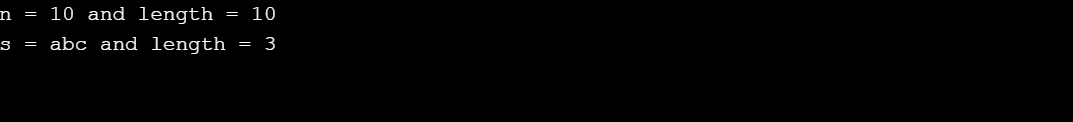### Structure Bindings

It basically allows you to declare multiple variables that are initialized with values from pairs, generic tuples or from custom structures and these multiples variable declarations happens in single statements.

Before C++17:

Before C++17, std::tie was used to declare multiple variables that are initialized with values from custom structures.

## C++

 `// Using tuple` `int` `a, b, c;` `std::tie(a, b, c) = std::make_tuple(1, 2, 3);`

When C++17:

Suppose you have a dictionary having names as keys and their favorite language as values and this is implemented using standard container map and you want to insert a new entry to it using insert method. This insert method returns an std::pair containing two pieces of information, the first item in the pair is an iterator and the second item is a boolean value.

## C++

 `// Below is the code to use` `// structure binding in C++17` `map fav_lang{` `    ``{ ``"John"``, ``"Java"` `},` `    ``{ ``"Alex"``, ``"C++"` `},` `    ``{ ``"Peter"``, ``"Python"` `}` `};`   `auto` `result` `   ``= fav_lang.insert({ ``"Henry"``,` `                       ``"Golang"` `});`

There are two cases to consider here:

• Whether new is not present in the dictionary or it is already present. If the new association (key-value pair) is not present in the dictionary, it gets inserted. So in this case, the returned pair contains an iterator pointing to the new element and the boolean value becomes True.
• If the new key is already present then the iterator points to the existing key and boolean value becomes False.

Now to write the code to inspect the boolean flag and insertion iterator, first write .first and .second to access elements in pair. C++ 17 can do better for this as:

• Using C++ 17 structure bindings to declare and initialize two variables with more meaningful names than first and second.
• Using the names position and success is much clearer than using first and second.
• The meaning of position and success is very straightforward i.e., position tells about where the iterator is and success tells whether the element is inserted or not.

Below is the program for the same:

## C++

 `// C++ 17 program to demonstrate ` `// Structure Bindings ` `  `  `#include ` `#include ` `#include ` `using` `namespace` `std; ` `  `  `// Driver Code ` `int` `main() ` `{ ` `    ``// Key-value pair declared inside ` `    ``// Map data structure ` `    ``map fav_lang{ ` `        ``{ ``"John"``, ``"Java"` `}, ` `        ``{ ``"Alex"``, ``"C++"` `}, ` `        ``{ ``"Peter"``, ``"Python"` `} ` `    ``}; ` `  `  `    ``// Structure binding concept used ` `    ``// position and success are used ` `    ``// in place of first and second ` `    ``auto``[process, success] ` `        ``= fav_lang.insert({ ``"Henry"``, ` `                            ``"Golang"` `}); ` `  `  `    ``// Check boolean value of success ` `    ``if` `(success) { ` `        ``cout << ``"Insertion done!!"` `             ``<< endl; ` `    ``} ` `  `  `    ``// Iterate over map ` `    ``for` `(``const` `auto` `& [ name, lang ] : fav_lang) { ` `  `  `        ``cout << name << ``":"` `             ``<< lang << endl; ` `    ``} ` `    ``return` `0; ` `}`

Output: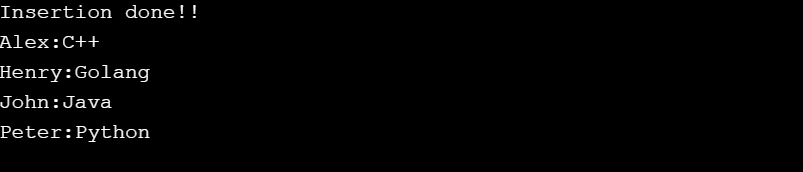### Folding Expressions

C++11 gave the option of variadic templates to work with variable number of input arguments. Fold expressions are a new way to unpack variadic parameters with operators. The syntax is as follows:

(pack op …)
(… op pack)
(pack op … op init)
(init op … op pack)
where pack represents an unexpanded parameter pack, op represents an operator and init represents a value.

• (pack op …): This is a right fold that is expanded like pack1 op (… op (packN-1 op packN)).
• (… op pack): This is a left fold that is expanded like ((pack1 op pack2) op …) op packN.
• (pack op … op init): This is a binary right fold that is expanded like pack1 op (… op (packN-1 op (packN op init))).
• (init op … op pack): This is a binary left fold that is expanded like (((init op pack1) op pack2) op …) op packN.

Before C++17:
To make a function that takes variable number of arguments and returns the sum of arguments.

## C++

 `// Below is the function that implements` `// folding expressions using variable` `// number of arguments`   `int` `sum(``int` `num, ...)` `{` `    ``va_list` `valist;`   `    ``int` `s = 0, i;`   `    ``va_start``(valist, num);` `    ``for` `(i = 0; i < num; i++)` `        ``s += ``va_arg``(valist, ``int``);`   `    ``va_end``(valist);`   `    ``return` `s;` `}`

When C++17:
To implement a recursive function like sum etc through variadic templates, this becomes efficient with C++17 which is better than C++11 implementations. Below is the template class of the same:

## C++

 `// Template for performing the` `// recursion using variadic template`   `auto` `C11_sum()` `{` `    ``return` `0;` `}`   `// Template Class` `template``<``typename` `T1, ``typename``... T>` `auto` `C11_sum(T1 s, T... ts)` `{` `    ``return` `s + C11_sum(ts...);` `}`

Below is the program to illustrate the same:

## C++

 `// C++ program to illustrate the` `// folding expression in C++17`   `#include ` `#include ` `using` `namespace` `std;`   `// Template Class` `template``<``typename``... Args>` `auto` `sum(Args... args)` `{` `    ``return` `(args + ... + 0);` `}`   `template` `<``typename``... Args>` `auto` `sum2(Args... args)` `{` `    ``return` `(args + ...);` `}`   `// Driver Code` `int` `main()` `{` `    ``// Function Calls` `    ``cout << sum(11, 22, 33, 44, 55)` `         ``<< ``"\n"``;` `    `  `    ``cout << sum2(11, 22, 33, 44, 55)` `         ``<< ``"\n"``;`   `    ``return` `0;` `}`

Output: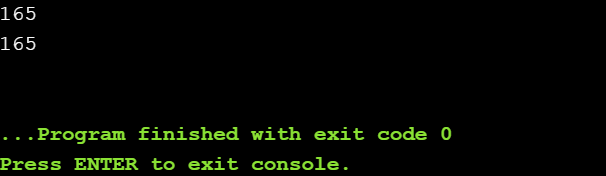### Direct list initialization of enums

In C++ 17 initialize initialization of enums using braces is allowed. Below is the syntax for the same:

enum byte : unsigned char {};
byte b {0}; // OK
byte c {-1}; // ERROR
byte d = byte{1}; // OK
byte e = byte{256}; // ERROR

Some of the library features of C++17:

• std::byte{b}: It is a unique type that applies the concept of byte as specified in the C++ language definition. A byte is a collection of bits and only bitwise operators can be used in this case. Below is the program to illustrate the same:

## C++

 `// Program to illustrate std::byte` `// in the C++ 17`   `#include ` `#include ` `using` `namespace` `std;`   `// Function to print byte a` `void` `Print(``const` `byte& a)` `{` `    ``cout << to_integer<``int``>(a) << endl;` `}`   `// Driver Code` `int` `main()` `{` `    ``byte b{ 5 };`   `    ``// Print byte` `    ``Print(b);`   `    ``// A 2-bit left shift` `    ``b <<= 2;`   `    ``// Print byte` `    ``Print(b);`   `    ``// Initialize two new bytes using` `    ``// binary literals` `    ``byte b1{ 0b1100 };` `    ``byte b2{ 0b1010 };` `    ``Print(b1);` `    ``Print(b2);`   `    ``// Bit-wise OR and AND operations` `    ``byte byteOr = b1 | b2;` `    ``byte byteAnd = b1 & b2;`   `    ``// Print byte` `    ``Print(byteOr);` `    ``Print(byteAnd);`   `    ``return` `0;` `}`

Output: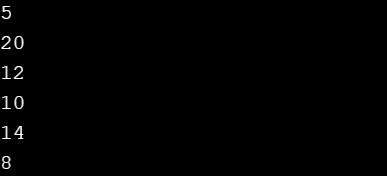• std::filesystem(): It provides a standard way to manipulate directories and files. In the below example a file a copied to a temporary path if there is available space. Below is the template for the same:

## C++

 `// For manipulating the file` `// directories`   `const` `auto` `FilePath {``"FileToCopy"``};`   `// If any filepath exists` `if``(filesystem::exists(FilePath)) {` `    ``const` `auto` `FileSize {` `         ``filesystem::file_size(FilePath)` `    ``};`   `    ``filesystem::path tmpPath {``"/tmp"``};`   `    ``// If filepath is available or not` `    ``if``(filesystem::space(tmpPath)` `                       ``.available > FileSize) {`   `       ``// Create Directory` `       ``filesystem::create_directory(` `                 ``tmpPath.append(``"example"``));`   `       ``// Copy File to file path` `       ``filesystem::copy_file(FilePath,` `                             ``tmpPath.append(``"newFile"``));` `    ``}` `}`

• std::apply(): Its parameters are a callable object which is to be invoked and a tuple whose elements need to be used as arguments. Below is the template for the same:

## C++

 `// Function that adds two numbers`   `auto` `add = [](``int` `a, ``int` `b) {` ` ``return` `a + b;` `};`   `apply(add, std::make_tuple(11, 22));`

• std::any(): The class any describes a type-safe container for single values of any type.The non-member any cast functions provide type-safe access to the contained object.

My Personal Notes arrow_drop_up
Recommended Articles
Page :